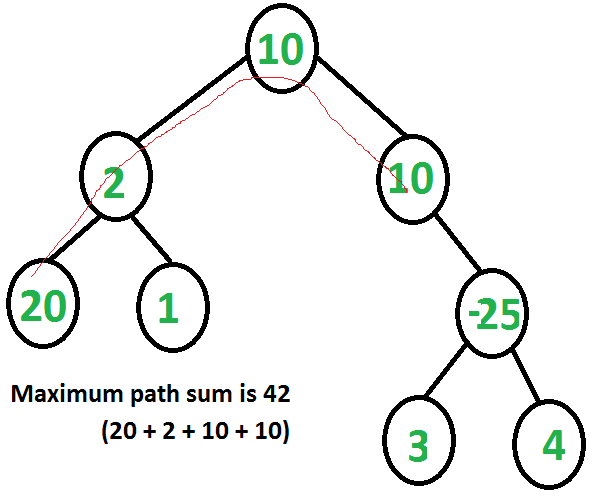# Maximum Path Sum Basic Information

suggest change

Maximum Path Sum is an algorithm to find out a path such that sum of element(node) of that path is greater than any other path.

For example, let’s we have a we a triangle as shown below.

```3
7   4
2   4   6
8   5   9   3```

In above triangle, find the maximum path which has maximum sum. Answer is, `3 + 7 + 4 + 9 = 23`

To find out the solution, as always we get an idea of Brute-Force method. Brute-Force method is good for this 4 rows triangle but think about a triangle with 100 or more than 100 rows. So, We can not use Brute-Force method to solve this problem.

Let’s move to dynamic programming approach.

Algorithm:

For each and every node in a triangle or in a binary tree there can be four ways that the max path goes through the node.

1. Node only
2. Max path through Left Child + Node
3. Max path through Right Child + Node
4. Max path through Left Child + Node + Max path through Right Child.

Example of Maximum Path Sum Algorithm:Space Auxiliary: `O(n)`

Time Complexity: `O(n)`